Matter Matter Anything that has mass and takes

• Slides: 16Matter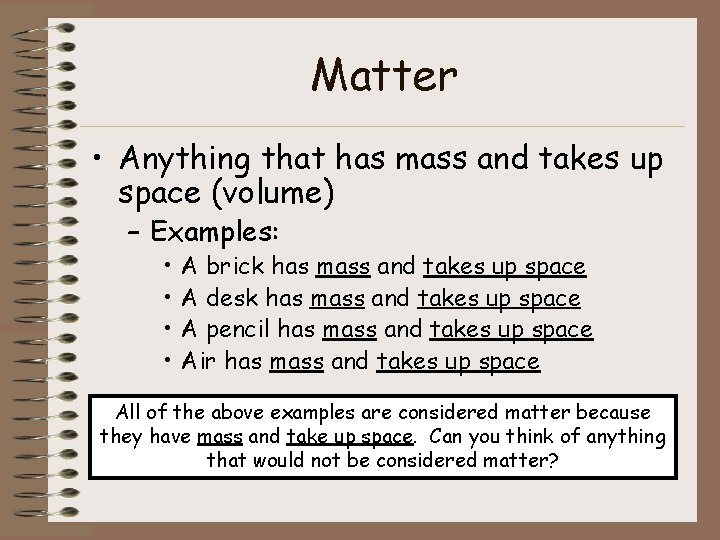Matter • Anything that has mass and takes up space (volume) – Examples: • • A brick has mass and takes up space A desk has mass and takes up space A pencil has mass and takes up space Air has mass and takes up space All of the above examples are considered matter because they have mass and take up space. Can you think of anything that would not be considered matter?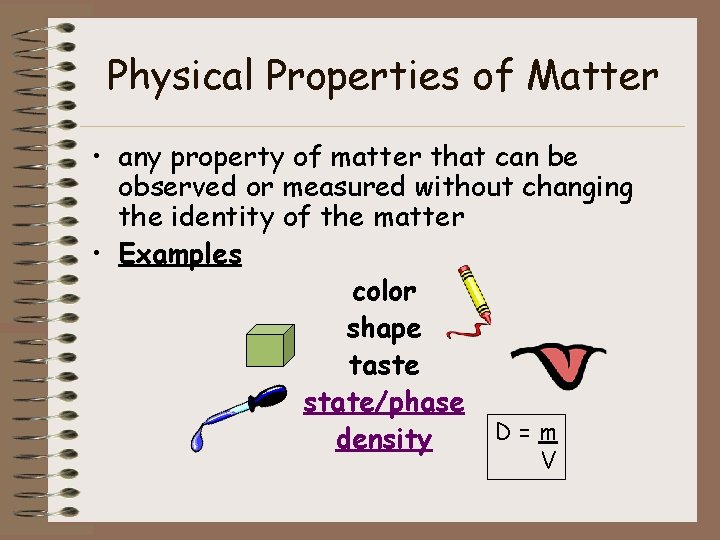Physical Properties of Matter • any property of matter that can be observed or measured without changing the identity of the matter • Examples color shape taste state/phase D=m density V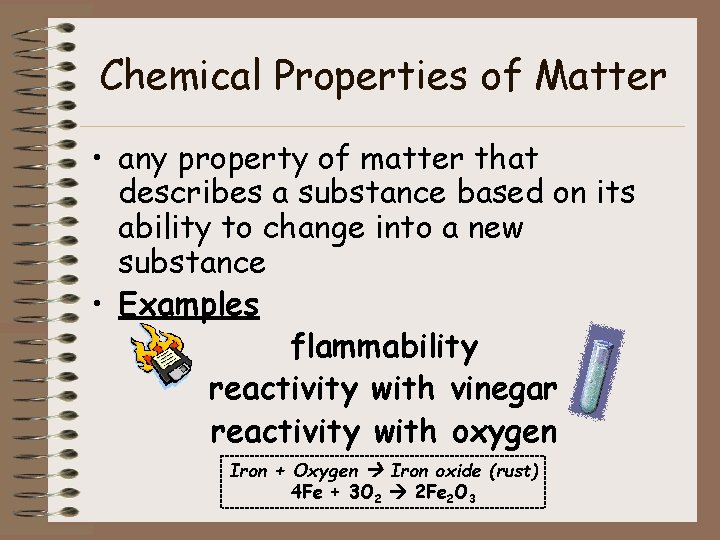Chemical Properties of Matter • any property of matter that describes a substance based on its ability to change into a new substance • Examples flammability reactivity with vinegar reactivity with oxygen Iron + Oxygen Iron oxide (rust) 4 Fe + 3 O 2 2 Fe 2 O 3Chemical or Physical Property? 1. Paper is white Physical Property 2. Boiling point of H 2 O is 100 o. C Physical Property 3. Zinc reacts with hydrochloric acid and creates hydrogen gas Chemical Property 4. Nitrogen does not burn Chemical Property 5. Sulfur smells like rotten eggs Physical PropertyComparing Physical and Chemical Properties Substance/Matter Physical Property Chemical Property Helium Less dense than air Nonflammable Hydrogen Less dense than air Flammable Wood Grainy texture Flammable Baking soda White powder Reacts with vinegar to produce bubbles Powdered sugar White powder Does not react with vinegar Rubbing alcohol Clear liquid Flammable Red food coloring Red color Reacts with bleach and loses color Iron Malleable Reacts with oxygen3 Physical States of Matter • Solid • Liquid • Gas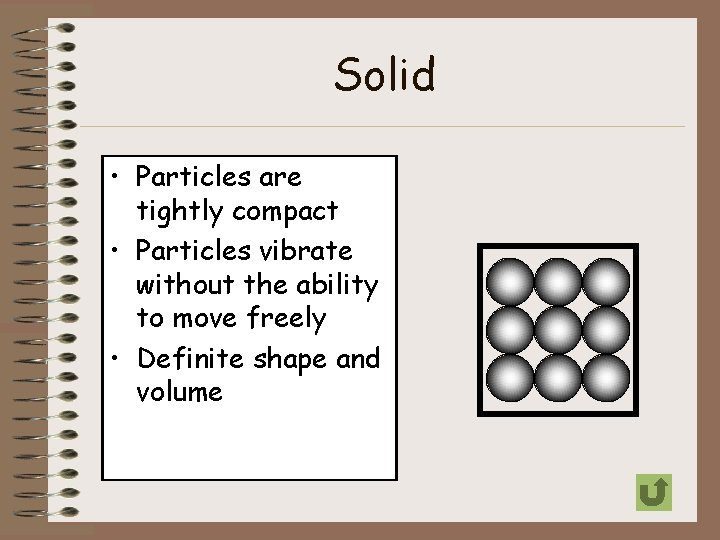Solid • Particles are tightly compact • Particles vibrate without the ability to move freely • Definite shape and volume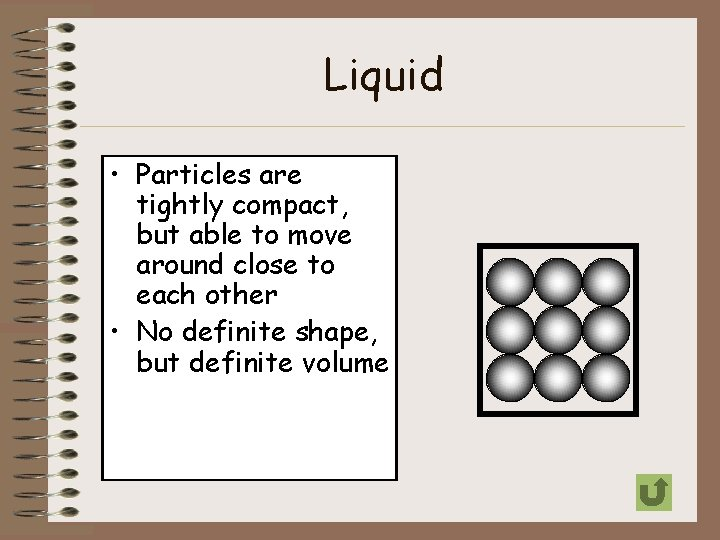Liquid • Particles are tightly compact, but able to move around close to each other • No definite shape, but definite volumeGas • Particles can easily spread out or move close together • Particles move freely and with a lot of energy • No definite shape or volumeEnergy and the States of Matter • The physical states of matter result from the amount of energy the particles composing the matter have. Basically, more energy means more movement for the particles and less energy means less movement. If you were to compare an ice cube and the steam created from boiling water, which would you think has more energy?States of Matter Continuum Taken from: http: //www. chem 4 kids. com/files/matter_becondensate. html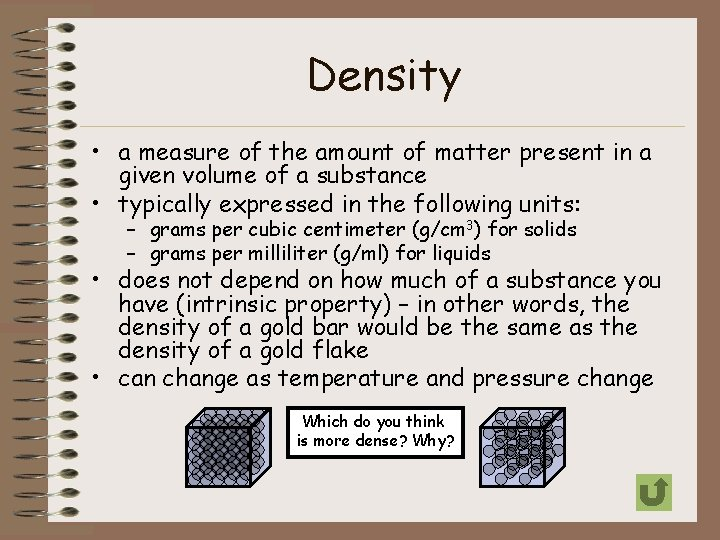Density • a measure of the amount of matter present in a given volume of a substance • typically expressed in the following units: – grams per cubic centimeter (g/cm 3) for solids – grams per milliliter (g/ml) for liquids • does not depend on how much of a substance you have (intrinsic property) – in other words, the density of a gold bar would be the same as the density of a gold flake • can change as temperature and pressure change Which do you think is more dense? Why?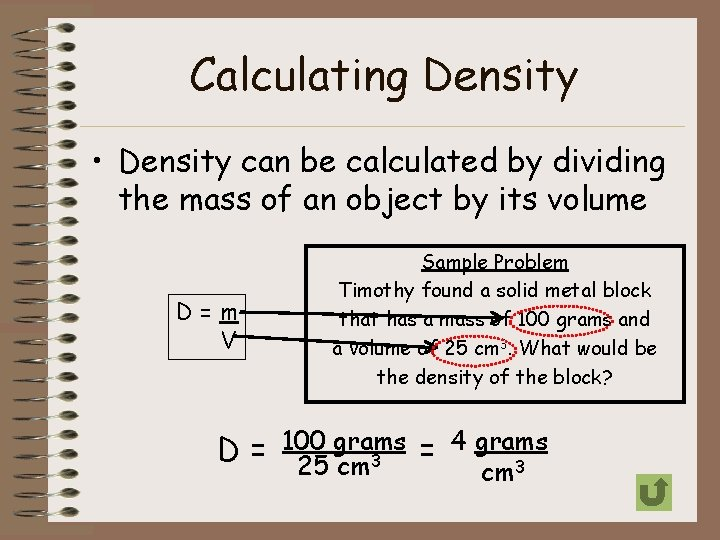Calculating Density • Density can be calculated by dividing the mass of an object by its volume D=m V Sample Problem Timothy found a solid metal block that has a mass of 100 grams and a volume of 25 cm 3. What would be the density of the block? grams = 4 grams D = 100 25 cm 3Practice Problems 1. Find the density of a substance with a mass of 27 g and a volume of 7 cm 3. D=m V 2. D = 27 g 7 cm 3 = 3. 86 grams cm 3 A block of maple has a mass of 20 grams and a volume of 26. 5 cm 3. What is the density of the block? D=m V D = 20 grams = 0. 75 grams 3 3 26. 5 cm cmThe Density Triangle D V=m m = D. V D V m D . V